#### Seeds: Random effect logistic regression

This example is taken from Table 3 of Crowder (1978), and concerns the proportion of seeds that germinated on each of 21 plates arranged according to a 2 by 2 factorial layout by seed and type of root extract. The data are shown below, where ri and ni are the number of germinated and the total number of seeds on the i th plate, i =1,...,N. These data are also analysed by, for example, Breslow: and Clayton (1993).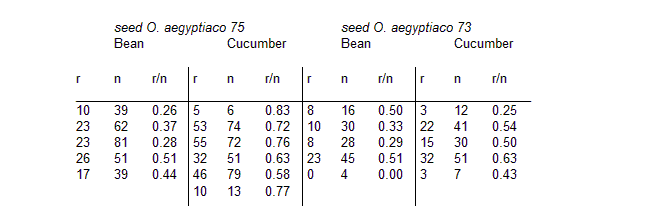The model is essentially a random effects logistic, allowing for over-dispersion. If pi is the probability of germination on the i th plate, we assume

ri ~ Binomial(pi, ni)

logit(pi) = α0 + α1x1i + α2x2i + α12x1ix2i + bi

bi ~ Normal(0, τ)

where x1i , x2i are the seed type and root extract of the i th plate, and an interaction term α12x1ix2i is included. α0 , α1 , α2 , α12 , τ are given independent "noninformative" priors.

Graphical model for seeds example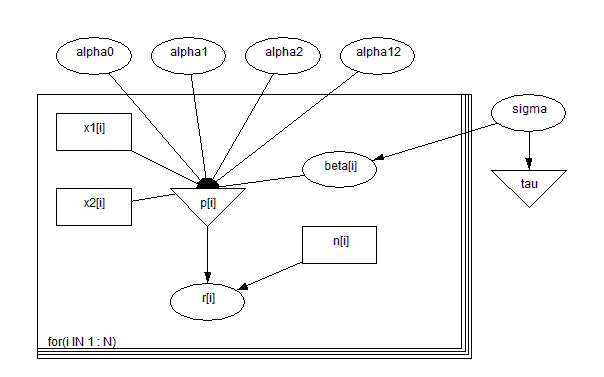BUGS language for seeds example

model
{
for( i in 1 : N ) {
r[i] ~ dbin(p[i],n[i])
beta[i] ~ dnorm(0.0,tau)
logit(p[i]) <- alpha0 + alpha1 * x1[i] + alpha2 * x2[i] +
alpha12 * x1[i] * x2[i] + beta[i]
}
alpha0 ~ dnorm(0.0,1.0E-6)
alpha1 ~ dnorm(0.0,1.0E-6)
alpha2 ~ dnorm(0.0,1.0E-6)
alpha12 ~ dnorm(0.0,1.0E-6)
sigma ~ dunif(0,10)
tau <- 1 / pow(sigma, 2)
}
##### Data
``` list(r = c(10, 23, 23, 26, 17, 5, 53, 55, 32, 46, 10, 8, 10, 8, 23, 0, 3, 22, 15, 32, 3),      n = c(39, 62, 81, 51, 39, 6, 74, 72, 51, 79, 13, 16, 30, 28, 45, 4, 12, 41, 30, 51, 7),      x1 = c(0, 0, 0, 0, 0, 0, 0, 0, 0, 0, 0, 1, 1, 1, 1, 1, 1, 1, 1, 1, 1),      x2 = c(0, 0, 0, 0, 0, 1, 1, 1, 1, 1, 1, 0, 0, 0, 0, 0, 1, 1, 1, 1, 1),      N = 21) ```
##### Inits for chain 1
``` list(alpha0 = 0, alpha1 = 0, alpha2 = 0, alpha12 = 0, sigma = 8) ```

##### Inits for chain 2
``` list(alpha0 = 0, alpha1 = 0, alpha2 = 0, alpha12 = 0, sigma = 1,beta = c(0, 0, 0, 0, 0, 0, 0, 0, 0, 0, 0, 0, 0, 0, 0, 0, 0, 0, 0, 0, 0)) ```
Results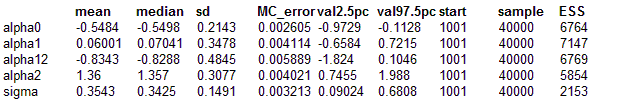We may compare simple logistic, maximum likelihood (from EGRET), penalized quasi-likelihood (PQL) Breslow and Clayton (1993) with the BUGS results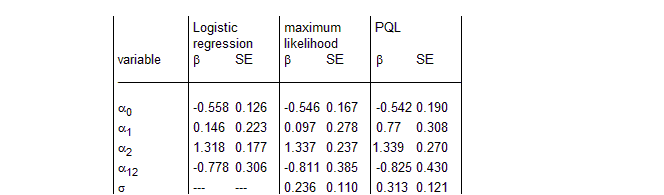Heirarchical centering is an interesting reformulation of random effects models. Introduce the variables

μi = α0 + α1x1i + α2x2i + α12x1ix2i

βi = μi + bi

the model then becomes

ri ~ Binomial(pi, ni)

logit(pi) = βi

βi ~ Normal(μi , τ)

The graphical model is shown below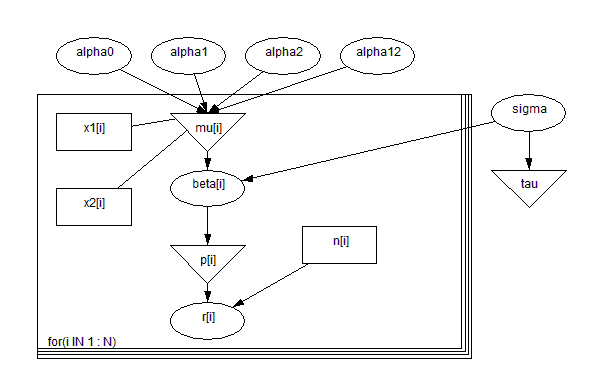model
{
for( i in 1 : N ) {
beta[i] ~ dnorm(mu[i], tau)
r[i] ~ dbin(p[i], n[i])
mu[i] <- alpha0 + alpha1 * x1[i] + alpha2 * x2[i] + alpha12 * x1[i] * x2[i]
logit(p[i]) <- beta[i]
}
alpha0 ~ dnorm(0.0, 1.0E-6)
alpha1 ~ dnorm(0.0, 1.0E-6)
alpha12 ~ dnorm(0.0, 1.0E-6)
alpha2 ~ dnorm(0.0, 1.0E-6)
sigma ~ dunif(0,10)
tau <- 1 / pow(sigma, 2)
}

Results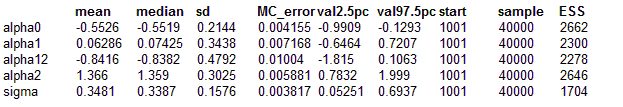This formulation of the model has two advantages: the squence of random numbers generated by the Gibbs sampler has better correlation properties and the time per update is reduced because the updating for the α parameters is now conjugate.